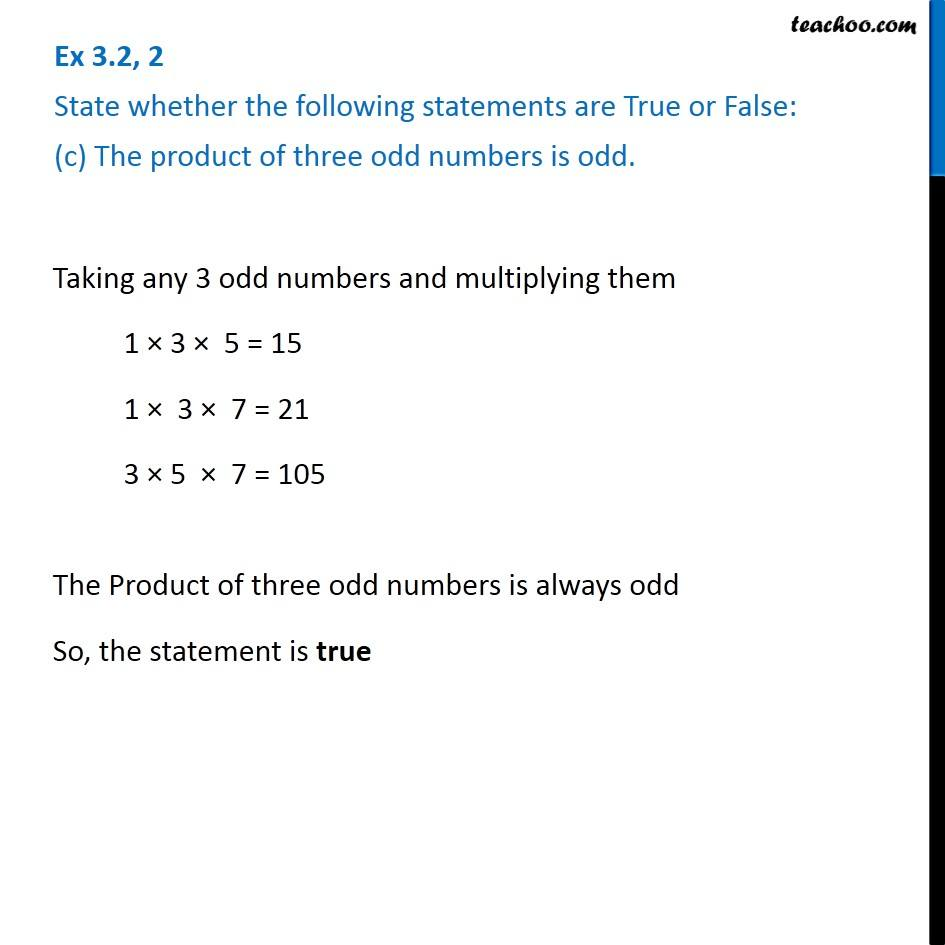Ex 3.2

Chapter 3 Class 6 Playing with Numbers
Serial order wiseLearn in your speed, with individual attention - Teachoo Maths 1-on-1 Class

### Transcript

Ex 3.2, 2 State whether the following statements are True or False: (c) The product of three odd numbers is odd. Taking any 3 odd numbers and multiplying them 1 × 3 × 5 = 15 1 × 3 × 7 = 21 3 × 5 × 7 = 105 The Product of three odd numbers is always odd So, the statement is true